2022 AL Rookie Odds
+200
2 to 1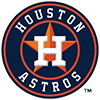Jeremy Pena
23.4% implied probability

+260
2.6 to 1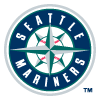Julio Rodriguez
19.5% implied probability

+650
6.5 to 1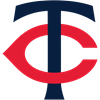Joe Ryan
9.4% implied probability

+700
7 to 1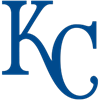Bobby Witt
8.8% implied probability

+1000
10 to 1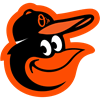Adley Rutschman
6.4% implied probability

+2000
20 to 1Steven Kwan
3.3% implied probability

+2200
22 to 1Spencer Torkelson
3.1% implied probability

+3500
35 to 1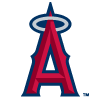Reid Detmers
2.0% implied probability

+3500
35 to 1George Kirby
2.0% implied probability

+5000
50 to 1MJ Melendez
1.4% implied probability

+5000
50 to 1Grayson Rodriguez
1.4% implied probability

+6000
60 to 1Triston Casas
1.2% implied probability

+6000
60 to 1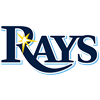Shane Baz
1.2% implied probability

+7000
70 to 1Riley Greene
1.0% implied probability

+8000
80 to 1Josh Lowe
0.9% implied probability

+9000
90 to 1Royce Lewis
0.8% implied probability

+10000
100 to 1Jose Siri
0.7% implied probability

+10000
100 to 1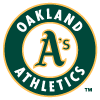Kevin Smith
0.7% implied probability

+10000
100 to 1Matt Brash
0.7% implied probability

+10000
100 to 1Cristian Pache
0.7% implied probability

+10000
100 to 1Daulton Jefferies
0.7% implied probability

+10000
100 to 1Gabriel Arias
0.7% implied probability

+10000
100 to 1Estevan Florial
0.7% implied probability

+10000
100 to 1Nick Pratto
0.7% implied probability

+10000
100 to 1Jake Burger
0.7% implied probability

+10000
100 to 1Nick Allen
0.7% implied probability

+10000
100 to 1Luis Gil
0.7% implied probability

+10000
100 to 1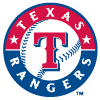Sam Huff
0.7% implied probability

+10000
100 to 1Jarren Duran
0.7% implied probability

+10000
100 to 1Richie Palacios
0.7% implied probability

+10000
100 to 1A.J. Alexy
0.7% implied probability

+10000
100 to 1Kyle Isbel
0.7% implied probability

+10000
100 to 1Xavier Edwards
0.7% implied probability

+10000
100 to 1Jordan Groshans
0.7% implied probability

+10000
100 to 1Vidal Brujan
0.7% implied probability

+10000
100 to 1Tyler Nevin
0.7% implied probability

+10000
100 to 1A.J. Puk
0.7% implied probability